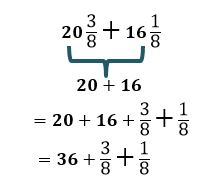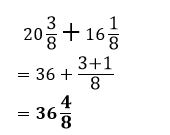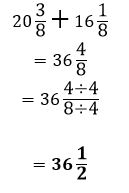# How to integrate fractions with common denominators

Want to find out how to integrate fractions with common denominators? We've provided a step-by-step guide of how to integrate fractions with common denominators to teach you everything you need to know.## Step 1: Add the numerators

Combine the fractions by adding the numerators directly since the denominators are already the same. So, if we want to add 8/15 and 2/15, we have

## Step 2: Simplify the fractions

Simplify the fractions by cancelling out common factors. Hence, when 8/15 and 2/15 are combined, the result would be equal to 2/3.

## Examples of how to integrate fractions with common denominators

Q1) Dan has sold 20 3/8 pounds of mangoes last week and 16 1/8 pounds of mangoes this week. How many pounds of mangoes were Dan able to sell for the past two weeks?

To find the total number of pounds of mangoes Dan was able to sell, we can add 20 3/8 and 16 1/8 pounds in total.

We can then add the whole number parts first.Since the fractions parts have the same denominators, we can add the numerators directly.We can then simplify the fraction by cancelling out common factors.This means that Dan was able to sell a total of 36 1/2 pounds of mangoes.

#### Join today

The fastest way to practice

Unlock our complete testing platform and improve faster that ever.